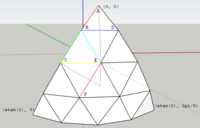# Optimization problem

#### BryanL

##### New member
Hi,
I am struggling to formulate the following problem.

Re the attached diagram, this is an n = 4 tiling of a face of an Icosahedron. The tiling is symmetric within the face and can be defined within the
light gray Schwarz triangle.

I want to be able to solve the six arcs AB, BC, ... , EF, with two equalities in the solution. E.g. arcs AB = BC, BC = CE. (The solution will be used to find all possible combinations of equalities).

The variables in this case are B theta and E theta. (phi of B, E is fixed by symmetry in this case although it wont be for all vertices for higher n).

I have all the spherical trig calculations and have previously solved this with blind iteration of the variables but for higher n it is impractical.

I know someone who has solved this but I only have hints : using Gauss-Newton, finite difference derivatives, Jacobian matix and a system of linear equtions.

I have read as much as I can on the Gauss-Newton method. Most references are fitting to a known data set. I thought I could see a way forward for one equality but can't see how to include the second equality.

Any help much appreciated.#### BryanL

##### New member
I managed to work it out (with a bit of guidance).
It is amazing how quick and powerful Gauss Newton is. In one iteration it found one equality correct to spreadsheet precision. In 4 iterations the sum of the residual squares was 0 and 5 iterations both equalities exactly.